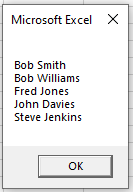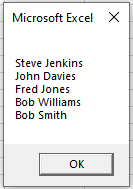# VBA Sort Array

This tutorial will demonstrate how to Sort values in an Array in VBA

## Sorting a One-Dimensional Array using a loop

Sorting an Array requires a bit of data manipulation using loops, variables and temporary arrays.

• You first need to populate the array with your values
• You then need to loop through the array twice!  Once to get a value from the current element array, and the while still in that loop, to get the value of the next element in the array.
• You then need to compare the elements – and move the 2nd one to the position of the first one if the 2nd one is alphabetically BEFORE the 1st one.

The example below demonstrates this procedure.

``````Sub SortAnArray()
Dim i As Long
'Set the array
Dim strName() As Variant
Dim Temp As Variant
'populate the array
strName() = Array("Bob Smith", "John Davies", "Fred Jones", "Steve Jenkins", "Bob Williams")
'loop through bound of the arry and get the first name
For i = LBound(strName) To UBound(strName) - 1
'loop through again, and check if the next name is alphabetically before or after the original
For j = i + 1 To UBound(strName)
If UCase(strName(i)) > UCase(strName(j)) Then
'if the name needs to be moved before the previous name, add to a temp array
Temp = strName(j)
'swop the names
strName(j) = strName(i)
strName(i) = Temp
End If
Next j
Next i
'Output the Array through a message box
MsgBox Join(strName(), vbCrLf)
End Sub``````

If you run this procedure, you would get the following message box.You can also sort the array in the other direction – eg: Z to A by changing this line of code

``If UCase(strName(i)) > UCase(strName(j)) Then``

to this line of code

``If UCase(strName(i)) < UCase(strName(j)) Then``

You would then get the following message box.Stop searching for VBA code online. Learn more about AutoMacro - A VBA Code Builder that allows beginners to code procedures from scratch with minimal coding knowledge and with many time-saving features for all users!